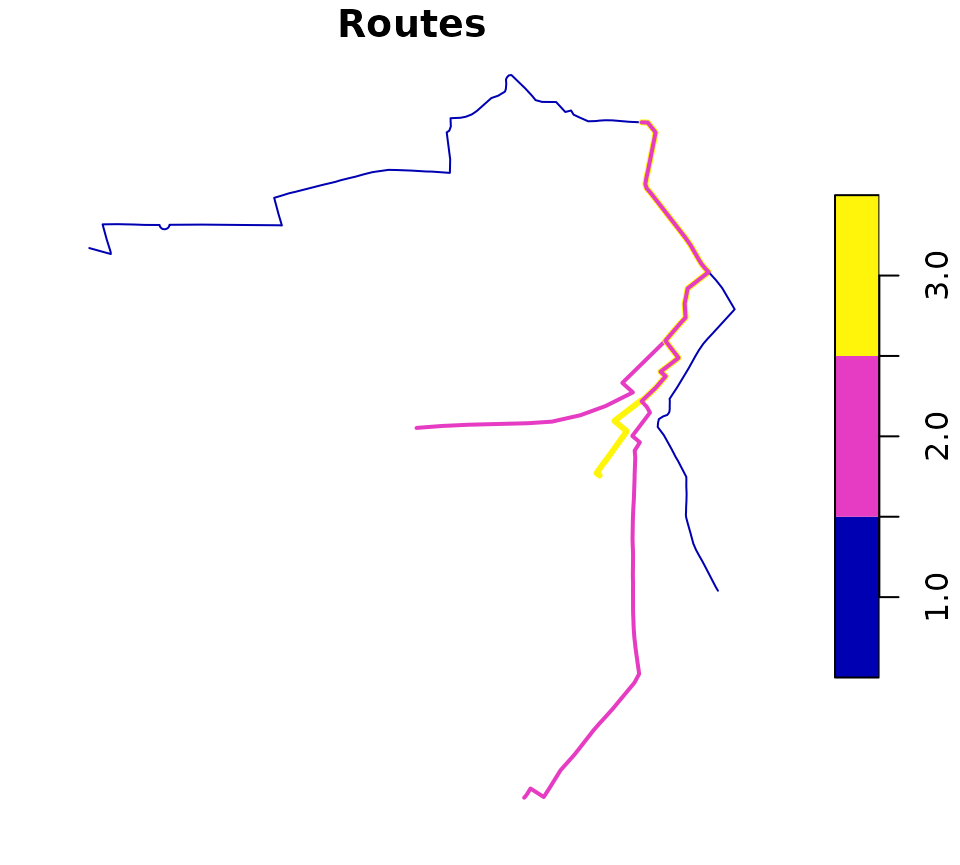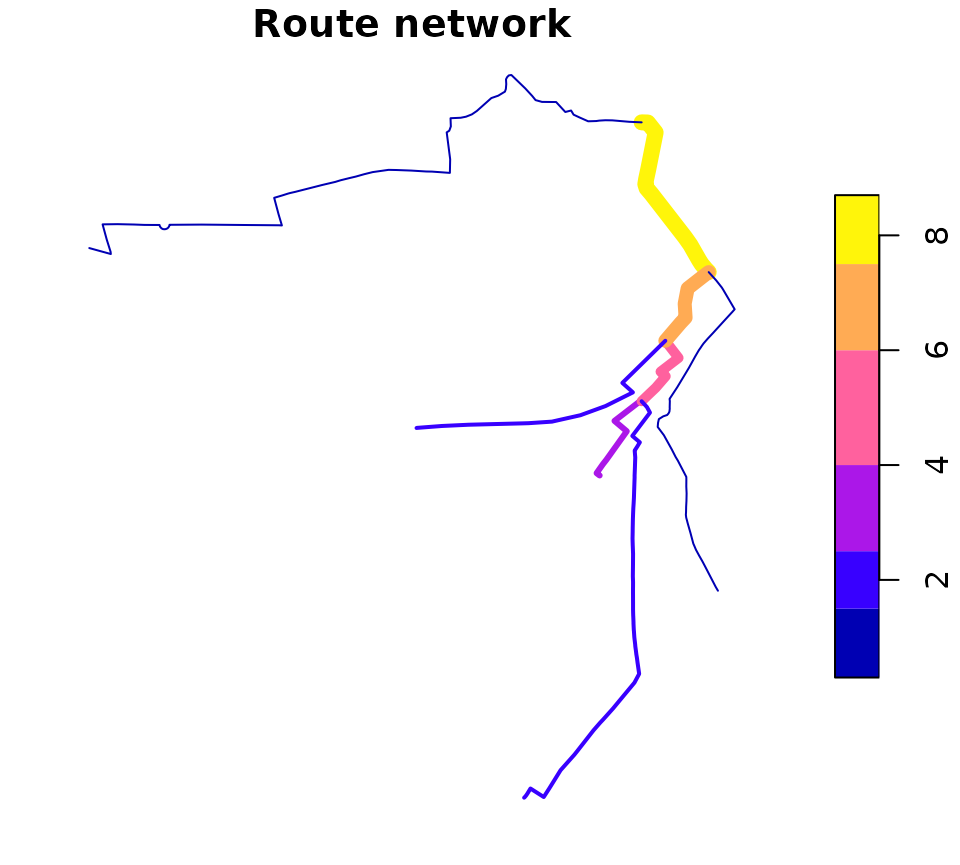``````library(stplanr)
library(sf)``````

## Introduction

Route networks represent the network of highways, cycleways, footways and other ways along which transport happens. You can get route network data from OpenStreetMap (e.g. via the `osmdata` R package) and other providers or transport network data.

## Creating route networks from overlapping routes

Unlike routes, each segment geometry in a route network can only appear once.

stplanr can be used to convert a series of routes into a route network, using the function `overline()`, as illustrated below:

``````library(stplanr)
library(sf)
sample_routes <- routes_fast_sf[2:6, 1]
sample_routes\$value <- rep(1:3, length.out = 5)
rnet <- overline(sample_routes, attrib = "value")
plot(sample_routes["value"], lwd = sample_routes\$value, main = "Routes")
plot(rnet["value"], lwd = rnet\$value, main = "Route network")``````The above figure shows how `overline()` breaks the routes into segments with the same values and removes overlapping segments. It is a form of geographic aggregation.

## Identifying route network groups

Route networks can be represented as a graph. Usually all segments are connected together, meaning the graph is connected. We can show that very simple network above is connected as follows:

``````touching_list = st_intersects(sample_routes)
igraph::is_connected(g)
#>  TRUE``````

A more complex network may not be connected in this way, as shown in the example below:

``````# piggyback::pb_download_url("r_key_roads_test.Rds")
touching_list = sf::st_intersects(rnet_disconnected)
igraph::is_connected(g)
#>  FALSE
sf:::plot.sfc_LINESTRING(rnet_disconnected\$geometry)``````The elements of the network are clearly divided into groups. We can identify these groups as follows:

``rnet_disconnected\$group = rnet_igroup(rnet_disconnected)``

## Routing on route networks

``````# plot(rnet\$geometry)
# xy_path <- sum_network_routes(sln = sln, start = xy_nodes, end = xy_nodes, sumvars = "length")
# # xy_path = sum_network_links(sln = sln, start = xy_nodes, end = xy_nodes)
# plot(rnet\$geometry)
# plot(xy_path\$geometry, add = TRUE, lwd = 5)``````

New nodes can be added to the network, although this should be done before the graph representation is created. Imagine we want to create a point half way along the the most westerly route segment in the network, near the coordinates -1.540, 53.826:

``````new_point_coordinates <- c(-1.540, 53.826)
p <- sf::st_sf(geometry = sf::st_sfc(sf::st_point(new_point_coordinates)), crs = 4326)``````

## Other approaches

Other approaches to working with route networks include:

• sDNA, an open source C++ library for analysing route networks and estimating flows at segments across network segments
• sfnetworks, an R package that provides an alternative igraph/sf spatial network class
• dodgr, an R package providing functions for calculating distances on directed graphs
• cppRouting, a package for routing in C++
• Chapter 10 of Geocomputation with R, which provides context and demonstrates a transport planning workflow in R.